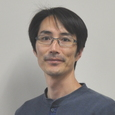## People

### Katsutoshi YAMANOIEmail yamanoi(@math.sci.osaka-u.ac.jp) Complex analysis, complex geometry Holomorphic curve, Nevanlinna theory, Kobayashi hyperbolicity

My research interest is Complex geometry and Complex analysis, both from the view point of Nevanlinna theory. In the geometric side, I am interested in the conjectural second main theorem in the higher dimensional Nevanlinna theory for entire holomorphic curves into projective manifolds. Also I am interested in the behavior of Kobayashi pseudo-distance of projective manifolds of general type. These problems are related to an algebraic geometric problem of bounding the canonical degree of algebraic curves in projective manifolds of general type by the geometric genus of the curves. In the analytic side, I am interested in classical problems of value distribution theory for meromorphic functions in the complex plane.